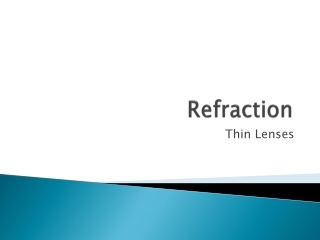DownloadDownload PresentationRefraction

# Refraction

Download Presentation## Refraction

- - - - - - - - - - - - - - - - - - - - - - - - - - - E N D - - - - - - - - - - - - - - - - - - - - - - - - - - -
##### Presentation Transcript

1. Refraction Thin Lenses

2. Types of Lenses • When light passes through a lens, it refracts twice • Once upon entering the lens and once upon leaving • Exiting ray is parallel to the entering light ray • Lens – a transparent object that refracts light rays causing them to converge or diverge to create an image • Two types of lenses • Converging – thicker in the middle than on the edges • Light rays converge to a single focal point • Diverging – thicker on the edges than in the middle • Light rays scatter

3. Types of Lenses • Both converging and diverging lenses have two focal points, one on each side of the lens • Both focal points have the same focal length • Focal length is measured by the distance from the focal point to the center of the lens • To draw ray diagrams, draw three rays • Parallel ray, central ray, and focal ray • Different for converging and diverging lenses

4. Types of Lenses

5. Characteristics of Lenses

6. Characteristics of Lenses

7. Characteristics of Lenses • Converging lenses can produce real or virtual lenses • Objects at infinity produce a point image at the focal point • Objects beyond 2F produce a small real image between F and 2F beyond the lens • Objects at 2F produce a real image of equal size at the 2F beyond the lens • Objects between F and 2F produce a larger real image beyond 2F on the other side of the lens • Objects at F do not produce an image • Objects inside F produce a larger virtual image on the same side of the lens

8. Characteristics of Lenses • Angular size – the apparent size of an object based on the size of the angle needed to view the entire object • Increases as you get closer to the object • As magnification increases, visible detail increases • Diverging lenses produce only virtual images on the same side of the lens • Smaller than object • Placement of the object does not matter

9. Characteristics of Lenses

10. The Thin-Lenses Equation and Magnification • The equations to find image distance and height for thin lenses are the same as those for mirrors • 1/p + 1/q = 1/f • M = h’/h = -q/p • Magnitude of magnification is greater than one when the image is larger than object • Magnitude of magnification is less than one when the image is smaller than object • Positive magnification is virtual image and upright • Negative magnification is real image and inverted

11. The Thin-Lens Equation and Magnification

12. Eyeglasses and Contact Lenses • Cornea – front of eyeball – acts as a converging lens directing light to the retina • Far-sighted – hyperopia – cornea focuses light behind the retina • Corrected with a converging lens • Cannot see near objects clearly • Near-sighted – myopia – cornea focuses light in front of the retina • Corrected with a diverging lens • Cannot see far objects clearly

13. Eyeglasses and Contact Lenses

14. Combinations of Thin Lenses • When using combinations of lenses, use one lens at a time • The image formed by the first object is treated as the object for the second lens and so on • To get overall magnification, multiply the magnifications of all lenses • Compound microscopes and refracting telescopes are combinations of converging lenses • Cannot use light microscopes to view atoms because the wavelengths of atoms are smaller than the wavelengths of visible light

15. Combinations of Thin Lenses

16. Combinations of Thin Lenses